Home Technical Mathematics Languages Science Social Science Art Literature Arabic Islamic Studies C.R.K History MusicVisual Art Clothing/Textile Home Management Shorthand
 Physics Paper 2, Nov/Dec. 2013
 Questions: 1 2 3 Main
Weakness/Remedies
Strength

QuestioN 2

The figure above shows a lagged calorimeter 50g of coarse iron filing.  The temperature of the calorimeter and its content was 25oC.

Water of mass M  =  20g at temperature 50oC was poured into the calorimeter and stirred.  The final temperature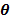of the mixture was noted and recorded.  The calorimeter was emptied, cleaned and dried.

With fresh and the same amount of iron fillings  each time, the process was repeated five more times, with M  = 30g,40g, 60g and 70g respectively, all at same temperature of 50oC

Fig. 2 shows the final temperature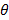i of the mixture, where= 1,2,3,4,5 and 6

1. Measure and record the real temperature;, of the mixtures.
2. In each case evaluate:(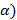xi=-  25o C;
(β)    yi  =  50oC – θ;
(γ)    Ti  =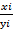.
(iv)        Plot a graph with Ti on the vertical axis and M on the horizontal axis.
(v)         Determine the slope, s, of the graph.
(vi)        State two precautions that are necessary to ensure accurate results when
performing this experiment.

(b) (i)         Explain the statement: ‘ the specific heat capacity of iron is 470 J kg-1 K-1’

(ii)        If  10s  =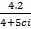determine the value of the specific heat capacity ci of iron.

This was a very popular question among the candidates and was fairly well attempted.  However, a few of the candidates did not observe the discontinuity in the scale drawing of the illustrated thermometer and so they did not obtain the correct raw reading of θ.  While some candidates were able to determine θ raw correctly ; a category of candidates were unable to convert it using the appropriate scale.  The other category were able to convert the raw θ correctly but failed while substituting θ convert to evaluate  xi, yi and Ti.  Some even confused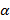, β and γ with x, y and T

Tabulation of readings, plotting of graphs and the determination of the slope was fairly well done.
Precautions and part (b) was poorly done. Very few candidates explained the expression “specific heat capacity of iron is 470 J kg-1K-1 correctly while majority of the responding candidates could not determine ci from the given equation.

_____________________________________________________________________________________________________

Candidates are expected to:

(i)         Record six values of M
(ii)        Measure and record six values of(iii)       Evaluate six values of x, y and T respectively
(v)         Plot  a graph with T on the vertical axis and M on the horizontal axis using
appropriate scales
(vi)       Distinguish between the axes and draw line of best fit
(vii)      Determine the slope of the graph
(viii)     State any two of the following precautions

1. Avoided parallax error in reading thermometer /weighing balance
2. Avoided thermometer bulb from touching the bottom/side of calorimeter
3. Stirred continuously / gently the mixture in the calorimeter
4. Noted /corrected zero error of weighing balance.
(x)   Determine the specific heat capacity C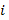from the equation 10s  =by